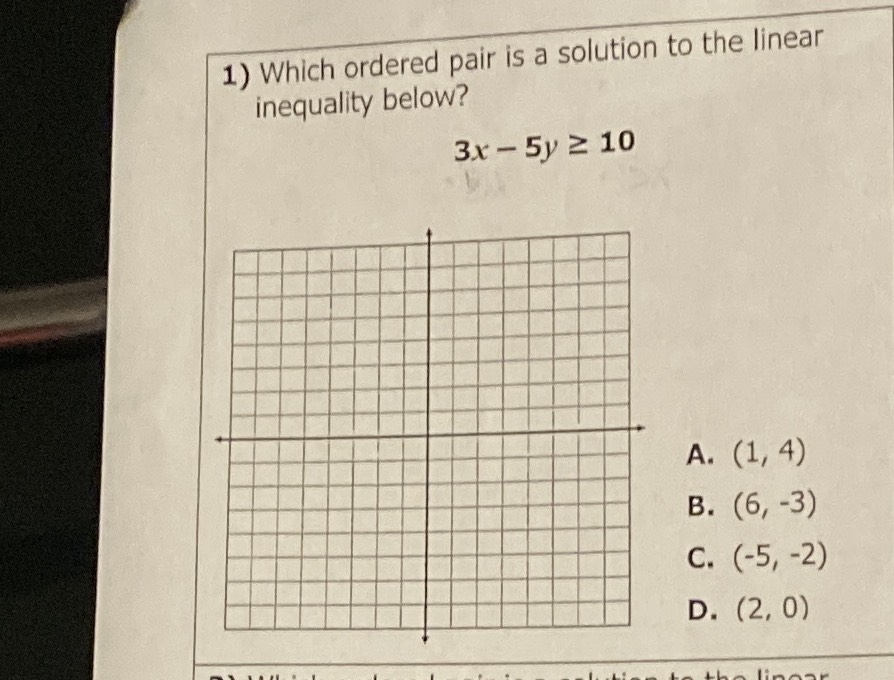### ¿Todavía tienes preguntas de matemáticas?

Pregunte a nuestros tutores expertos
Algebra
Pregunta1) Which ordered pair is a solution to the linear inequality below?

$$3 x - 5 y \geq 10$$

A. $$( 1,4 )$$

B. $$( 6 , - 3 )$$

C. $$( - 5 , - 2 )$$

D. $$( 2,0 )$$

B. $$( 6 , - 3 )$$